### Day 06 - Continuity and One-sided Limits - 08.24.15

Update

Bell Ringer

1. Find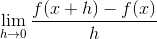if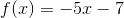.

1. 0

2. undefined

3. 5

4. -5

5. none of the above

2. Findif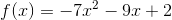.

1. undefined

2. -14x

3. 14x

4. -14x - 9

5. none of the above

3. Find the x-values (if any) at which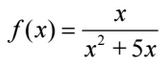is not continuous.

1. f(x) is continuous for all real x.

2. f(x) is not continuous at x = 0, –5 and both the discontinuities are nonremovable.

3. f(x) is not continuous only at x = –5 and f(x) has a removable discontinuity at x = –5 .

4. f(x) is not continuous only at x = 0 and f(x) has a removable discontinuity at x = 0 .

5. f(x) is not continuous at x = 0, –5 and f(x) has a removable discontinuity at x = 0 .

4. Find the x-values (if any) at which the function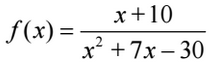is not continuous. Which of the discontinuities are removable?

1. no points of discontinuity.

2. x = –10 (not removable), x = 3 (removable)

3. x = –10 (removable), x = 3 (not removable)

4. no points of continuity.

5. x = –10 (not removable), x = 3 (not removable)

5. Find the limit (if it exists).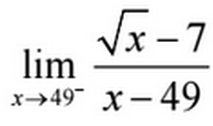1. 1 / 14

2. 0

3. 1 / 98

4. -1 / 14

5. limits does not exist

Review
• Math Overview (video)
• Numbers
• Relationships
• Shapes
• Change
• Limits
• Intro to Limits (video)
• Nonexistent Limits (video)
• How are limits found numerically and graphically? (checkpoints)
• How are limits found algebraically? (checkpoints)

Lesson
• Challenge 3
• Find a that makes the following function continuous.
•• Find the following limit:
• Graph
• Continuity (videoand One-sided Limits (video)

Exit Ticket
• Posted on the board at the end of the blockHomework
• WNQ
• Last section of Unit 1
Lesson Objectives
• How can discontinuity of a function be described?
• How are one-sided limits related to regular limits?

#### In-Class Help Requests

Standard(s)
• APC.2
• Define and apply the properties of limits of functions.
• Limits will be evaluated graphically and algebraically.
• Includes:
• ​limits of a constant
• ​limits of a sum, product, and quotient
• ​one-sided limits
• ​limits at infinity, infinite limits, and non-existent limits*
• APC.3
• Use limits to define continuity and determine where a function is continuous or discontinuous.
• Includes:
• ​continuity in terms of limits
• continuity at a point and over a closed interval
• ​application of the Intermediate Value Theorem and the Extreme Value Theorem
• ​geometric understanding and interpretation of continuity and discontinuity